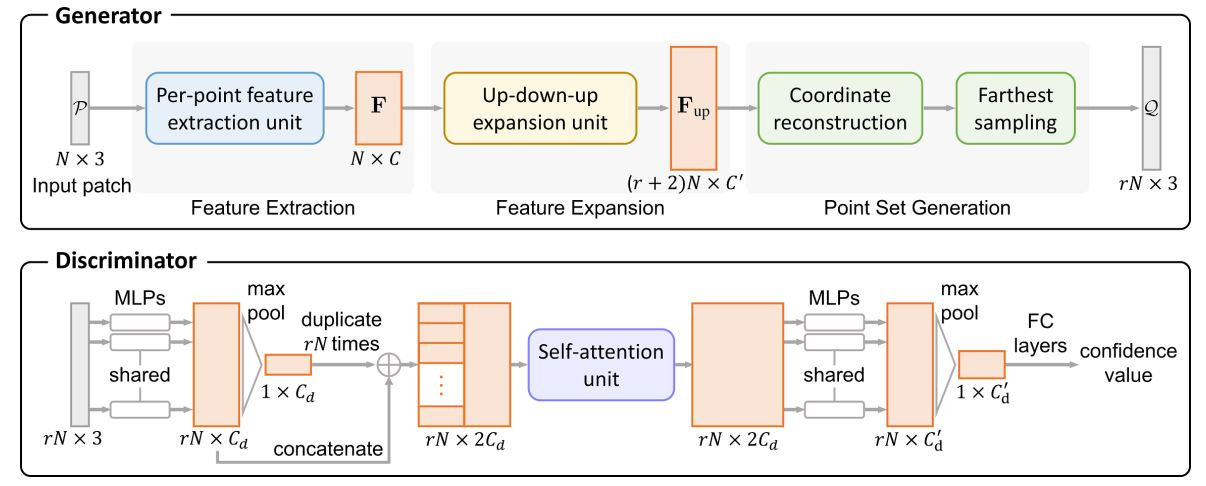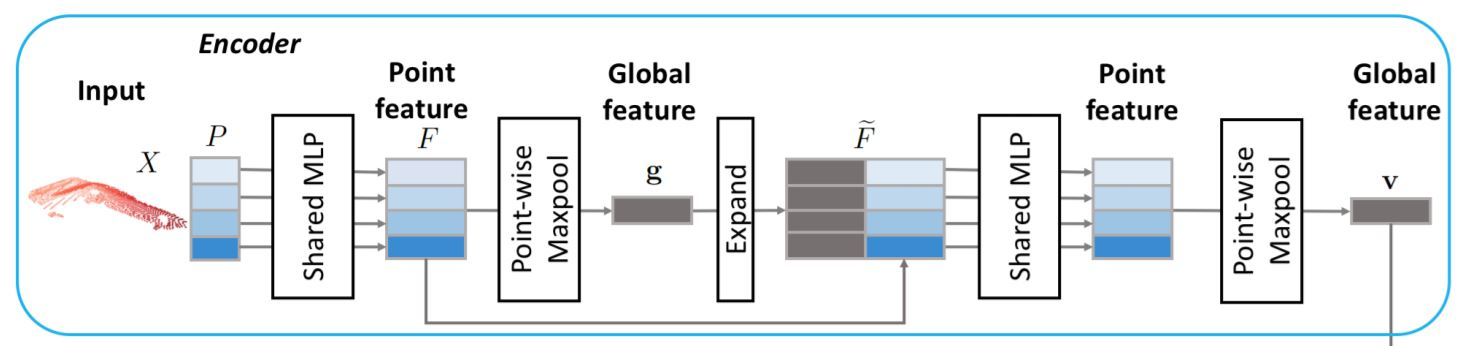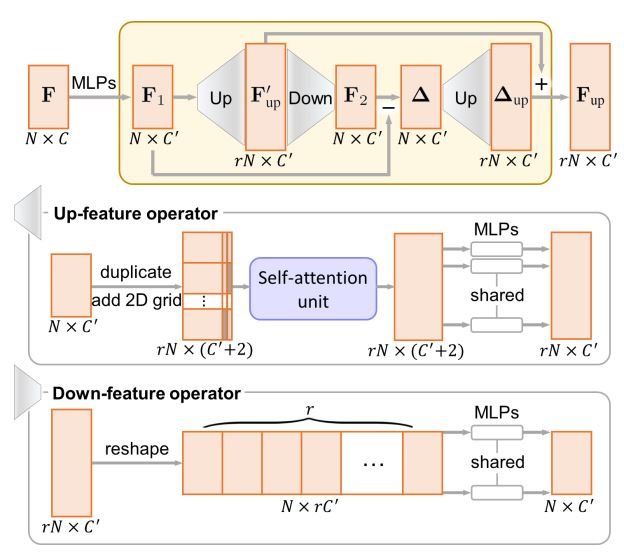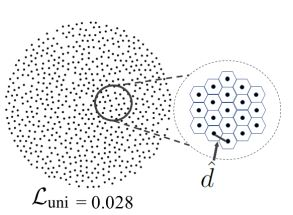ICCV2019 PU-GAN

## 参考论文

PU-GAN: a Point Cloud Upsampling Adversarial Network

## Method

### Overview

1. 输出Q与输入P应该对一个目标物体描述了同样的集合特征，因此在集合Q中的点应该分布并且覆盖在目标物体的表面。

2. 即使输入P中的点是稀疏并且不均匀的，输出Q上的点也应该均匀分布在目标物体的表面

PU-GAN的网络结构如下所示：### 网络结构 Network Architecture

#### 生成器 Generator#### 判别器 Discriminator### Up-down-up Expansion Unit

Up-down-up Expansion Unit结构图如下所示：### Self-attention Unit$W = f_{softmax}(G_TH)$

## Loss function

$\mathcal{L}_{gan}(G) = \frac{1}{2}[D(\mathcal{Q}) - 1]^2$

$\mathcal{L}_{gan}(D) = \frac{1}{2}[D(\mathcal{Q})^2 + (D(\hat{\mathcal{Q}}) - 1)^2]$

### Uniform loss

$U_{imbalance}(S_j) = \frac{(|S_j| - \hat{n})^2}{\hat{n}}$$U_{clutter}(S_j) = \sum_{k=1}^{|S_j|}\frac{(d_{j,k}-\hat{d})^2}{\hat{d}}$

$\mathcal{L}_{uni} = \sum_{j=1}^{M}U_{imbalance}(S_j)\cdot U_{clutter}(S_j)$

### Reconstruction loss

$\mathcal{L}_{rec} = \min_{\phi : \mathcal{Q} \to \hat{\mathcal{Q}}} \sum_{q_i \in \mathcal{Q}} ||q_i - \phi (q_i)||_2$

### Compound loss

$\mathcal{L}_G = \lambda_{gan}\mathcal{L}_{gan}(G) + \lambda_{rec}\mathcal{L}_{rec} + \lambda_{uni}\mathcal{L}_{uni}$

$\mathcal{L}_D = \mathcal{L}_{gan}(D)$